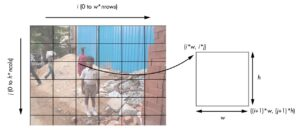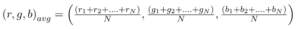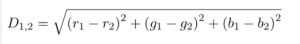# Implementing Photomosaics

Introduction

A photomosaic is an image split into a grid of rectangles, with each replaced by another image that matches the target (the image you ultimately want to appear in the photomosaic). In other words, if you look at a photomosaic from a distance, you see the target image; but if you come closer, you will see that the image actually consists of many smaller images. This works because of the how the human eye works.

There are two kinds of mosaic, depending on how the matching is done. In the simpler kind, each part of the target image is averaged down to a single color. Each of the library images is also reduced to a single color. Each part of the target image is then replaced with one from the library where these colors are as similar as possible. In effect, the target image is reduced in resolution (by downsampling), and then each of the resulting pixels is replaced with an image whose average color matches that pixel.

In the more advanced kind of photographic mosaic, the target image is not downsampled, and the matching is done by comparing each pixel in the rectangle to the corresponding pixel from each library image. The rectangle in the target is then replaced with the library image that minimizes the total difference. This requires much more computation than the simple kind, but the results can be much better since the pixel-by-pixel matching can preserve the resolution of the target image.

How to create Photomosaics?

1. Read the tile images, which will replace the tiles in the original image.
2. Read the target image and split it into an M×N grid of tiles.
3. For each tile, fnd the best match from the input images.
4. Create the fnal mosaic by arranging the selected input images in an M×N grid.

Splitting the images into tilesNow let’s look at how to calculate the coordinates for a single tile from this grid. The tile with index (i, j) has a top-left corner coordinate of (i*w, i*j) and a bottom-right corner coordinate of ((i+1)*w, (j+1)*h), where w and h stand for the width and height of a tile, respectively. These can be used with the PIL to crop and create a tile from this image.

Averaging Color Values

Every pixel in an image has a color that can be represented by its red, green, and blue values. In this case, you are using 8-bit images, so each of these components has an 8-bit value in the range [0, 255]. Given an image with a total of N pixels, the average RGB is calculated as follows:Matching Images

For each tile in the target image, you need to fnd a matching image from the images in the input folder specifed by the user. To determine whether two images match, use the average RGB values. The closest match is the image with the closest average RGB value.
The simplest way to do this is to calculate the distance between the RGB values in a pixel to fnd the best match among the input images. You can use the following distance calculation for 3D points from geometry:Now lets try to code this out

```#Importing the required libraries
import os, random, argparse
from PIL import Image
import imghdr
import numpy as np

def getAverageRGBOld(image):
"""
Given PIL Image, return average value of color as (r, g, b)
"""
# no. of pixels in image
npixels = image.size*image.size
# get colors as [(cnt1, (r1, g1, b1)), ...]
cols = image.getcolors(npixels)
# get [(c1*r1, c1*g1, c1*g2),...]
sumRGB = [(x*x, x*x, x*x) for x in cols]
# calculate (sum(ci*ri)/np, sum(ci*gi)/np, sum(ci*bi)/np)
# the zip gives us [(c1*r1, c2*r2, ..), (c1*g1, c1*g2,...)...]
avg = tuple([int(sum(x)/npixels) for x in zip(*sumRGB)])
return avg

def getAverageRGB(image):
"""
Given PIL Image, return average value of color as (r, g, b)
"""
# get image as numpy array
im = np.array(image)
# get shape
w,h,d = im.shape
# get average
return tuple(np.average(im.reshape(w*h, d), axis=0))

def splitImage(image, size):
"""
Given Image and dims (rows, cols) returns an m*n list of Images
"""
W, H = image.size, image.size
m, n = size
w, h = int(W/n), int(H/m)
# image list
imgs = []
# generate list of dimensions
for j in range(m):
for i in range(n):
# append cropped image
imgs.append(image.crop((i*w, j*h, (i+1)*w, (j+1)*h)))
return imgs

def getImages(imageDir):
"""
given a directory of images, return a list of Images
"""
files = os.listdir(imageDir)
images = []
for file in files:
filePath = os.path.abspath(os.path.join(imageDir, file))
try:
# explicit load so we don't run into resource crunch
fp = open(filePath, "rb")
im = Image.open(fp)
images.append(im)